# Numerical and Experimental Computation of Airflow in a Transport Container

Numerical and Experimental Computation of Airflow in a Transport Container

T. CardinaleP. De Fazio F. Grandizio

ENEA, Italian National Agency for New Technologies, Energy and Sustainable Economic Development, Trisaia R. C., DTE – BBC unit, ss 106 Jonica km. 419,55, Rotondella (MT), Italy

Corresponding Author Email:
tiziana.cardinale@enea.it
Page:
S323-S331
|
DOI:
https://doi.org/10.18280/ijht.34S219
|
Accepted:
|
Published:
31 October 2016
| Citation

OPEN ACCESS

Abstract:

The aim of this work is to perform a numerical and experimental analysis of convective flows inside an intermodal container refrigerated in hybrid mode, active by compressor and passive by eutectic plates, in the course of a simulated trip, in order to verify the distribution uniformity of the cold. This report shows the numerical simulation model implemented thanks to the use of Comsol Multiphysics 4.3b, a scientific commercial software CFD (Computational Fluid Dynamics) that allows thermofluidodynamic simulations. Two operation modes were considered in the model, one in laminar flow to reproduce the OFF cycle of the compressor with the only action of the eutectic plates, the other one in turbulent flow to reproduce the ON cycle of the compressor. In the second case, the k-ε model was used as a model of turbulence RANS (Reynolds Averaged Navier Stokes). The results of this numerical analysis were compared with experimental data acquired during the simulated trip to validate the implemented model.

Keywords:

CFD, Model, Convective flows, Air distribution, Hybrid refrigeration.

1. Introduction

The transport of fresh and cold products within refrigerated containers involves the maintenance of the cold chain, useful to ensure the quality of products itself. The value of temperature and the uniform distribution within refrigerated containers are directly related to the trend of the air flows, phenomenon influenced by several interdependent parameters that act simultaneously and make the problem quite complex.

Numerical modelling tool represents a viable solution to this physical phenomenon, although experimental data for its validity are also necessary.

In recent years Computational Fluid Dynamics (CFD) methodology, based on solving the flow equations (continuity equation, Navier-Stokes equations, energy conservation), has become widely used for the development of fluid flow problems. The numerical solution involves an iterative process divided into 3 phases: pre-processing with the definition of the operating parameters, solving with the calculation of the converged solution for a fixed accuracy and post-processing with the visualization and analysis of the temperature and velocity maps.

As part of a research project financed with national funds, researchers from the DTE-BBC ENEA Trisaia laboratory have made numerical and experimental analysis of convective flows inside the useful volume of an intermodal mobile container refrigerated in hybrid mode, active with the compressor and passive with eutectic plates.

In order to verify the uniformity of the distribution of the cold inside the container, during the simulation of an intermodal trip, experimental data have been before acquired and a numerical model has been subsequently developed by means of the CFD Comsol Multiphysics 4.3 software.

The comparison between the experimental results and the simulations of the implemented model has allowed its validation.

2. Experimental Tests

2.1 Characteristics of the hybrid refrigerated container

The sketch of the mobile container refrigerated by an active-passive hybrid system, active with the compressor and passive with eutectic plates, is shown in Figure 1 with the known geometric dimensions. The container consists of insulating sandwich panels composed of fiberglass laminates, with a transmittance value equal to 0.34 (W/m2 K).

The inlet and outlet sections of the air flow introduced from the compressor are positioned on the same side in front of the container. The flow velocity of the cold airflow is equal to 15 m/s, while the volume of the air flow is equal to 5000 m3/h.

## figure_1.png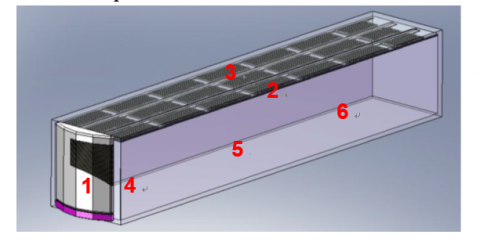Figure 1. Schematization of container and PT100

Starting from available data, the sensors for temperature measurement are placed in various areas of the container . In detail, 6 PT100 probes (2 for eutectic and 4 for the walls) have allowed to monitor the temperature in the same number of internal points, limit imposed by the capacity of the acquisition instrument inside the container.

The 6 probes have been placed as in Figure 1: the probes 4-5-6 equally spaced along the side wall of the container at a height equal to 50% of the total one, the probes 2-3 in correspondence of the central elements of the eutectic plates, the probe 1 in proximity of the grid on the compressor wall.

The monitoring of the temperatures inside of the container has occurred with an acquisition rate equal to 10 minutes for the entire duration of an intermodal trip (train, tire, ship) simulated, in cold regime, passing through the different operating modes (active or passive) according to the different phases of the travel. The data acquisition from the probes has been also performed from the charging phase of the eutectic plates, before the start of the simulated trip, until the opening of the doors at the end of trip for a total of 79 h. During the entire simulated trip the compressor started to recharge the plates when the internal temperature increased up to -22 °C and terminated when the internal temperature dropped to -24 °C, with a previously set threshold value equal to 2 °C. To ensure the above thermal conditions, the compressor has worked for approximately 50% of the time, with cycles of about 20’ ON e 20’ OFF.

2.2 Results of experimental tests

Temperatures were acquired by the probes from 7:30 on the 1st day until 14:30 of the 4th day.

The temperature T1 (Figure 2) and the temperature T4 (Figure 3) were quite similar as acquired from 2 probes positioned close to the wall of the compressor. It is evident the oscillation of the temperature due to the ON-OFF cycles of the compressor of about 2 °C, slightly higher for the T1 probe that is closer to the compressor than the T4 probe. The temperature range is approximately between the -24 °C (ON) and the -22 °C (OFF). The detected average temperature is equal to -23 °C on regime. The T2-T3 temperatures, relating to eutectic plates, are in a range between -28 °C and -27 °C, with oscillation of 1/2 °C due to the switch-on of the compressor and then to the ON-OFF charging of the plates with a rate of 20 minutes (Figure 4). The T5 probe (Figure 5) recorded the temperatures in the central part of the mobile container reporting an average temperature of about -23.5 °C and a difference of just over 1 °C between the compressor switch-on phase (-24.2 °C) and the switch-off one (-23 °C). The temperature recorded by the probe T6 (Figure 6) placed close to the doors has had a variation of approximately 1 °C and has changed from -22.3 °C (OFF) to -23.2 °C (ON) with an average temperature -22.7 °C.

By examining the differences between the temperatures recorded by the probes T4, T5, and T6 with the compressor off and on, in the ON phase the coldest area would seem the central one, then the compressor one and, finally, the area near the doors, while for the OFF phase the coldest area always remains the central one, while the two opposite areas (compressor and doors) are equal. In the totality of the ON-OFF functioning of the compressor, the analysis of average temperatures in the three areas detected by the probes, highlights that, in a bottom-up temperature scale, the central area has the lowest temperature, then the compressor area, finally the doors area (Table 1).

## figure_2.png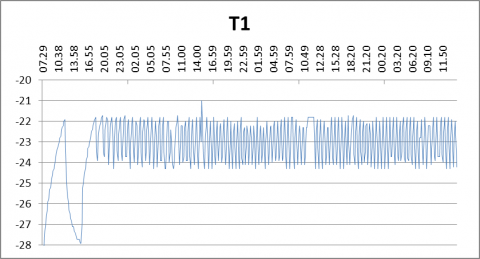Figure 2. T1 probe temperature trend

## figure_3.png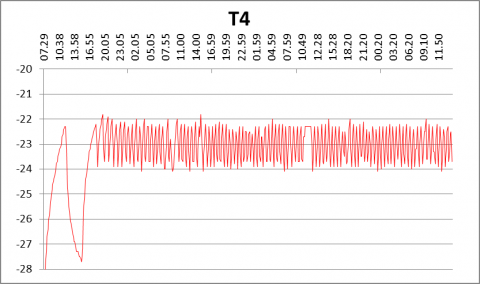Figure 3. T4 probe temperature trend

## figure_4.png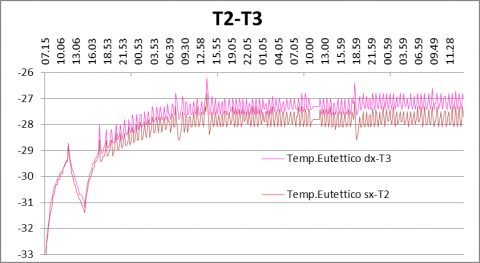Figure 4. T2 e T3 probes temperature trend

## figure_5.png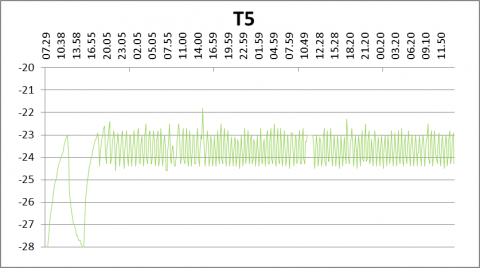Figure 5. T5 probe temperature trend

## figure_6.png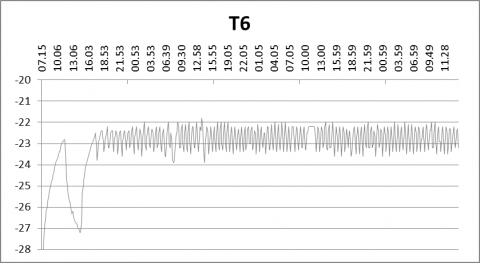Figure 6. T6 probe temperature trend

Table 1. T4, T5 and T6 during ON-OFF phases

 Temperatures ON OFF T4 -23.8°C -22.2°C T5 -24.2°C -23°C T6 -23.2°C -22.3°C
3. Numerical Modelling

3.1 Comsol Multiphysics 4.3

The numerical analysis was implemented with the aid of Comsol Multiphysics 4.3b, a scientific-commercial CFD software, which enables multiphysics simulations within the same model, based on partial differential equation (PDE) through the use of the finite element method (FEM) .

The operational scheme for the use of Comsol Multiphysics 4.3b initially provides the choice of the simulation spatial dimension. Then the physics of theoretical model has been implemented. Before proceeding with data entry and with the assignment of boundary conditions and initial ones, the last step is to decide the type of study that you want to lead, stationary or time-dependent.

In this case study, initially a 3D real geometry complete of walls, compressor, eutectic plates, doors, has been chosen as the spatial dimension of the container (Figure 5).

## figure_7.pngFigure 7. 3D geometry of the container

Considered the long times of the simulation model calculation, due to the considerable real dimensions of the container, we have opted for a workspace with a 2D spatial dimension z-x.

In summary, therefore, we defined:

- Geometry of the model, which corresponds to the actual physical size of the container.

- Materials with their domains (air, sandwich panel, eutectic).

- Non-Isothermal Flow as a type of physics.

- Mesh depending on the model geometry.

- Study time-dependent.

The geometry has been defined according to the known parameters using the Polygon function which allows to build the geometry as a sequence of connected line segments. Then the Cartesian coordinate system has been defined.

In general, the container was represented as shown in Figure 8; the entry point (I-Inlet), the output point (O-Outlet) and outer walls (Wall) are highlighted. So we have a two-dimensional space, 7 domains and 29 boundaries.

In the definition of the model, with the expression Non-isothermal flow (nift) we refer to the fluid flows with temperatures that are not constant. When a fluid is subjected to a change of temperature, the material properties, such as density and viscosity, change accordingly.

3.2 Laminar flow

An empty container model in laminar flow has been reproduced to simulate OFF cycle of the compressor with the only action of the cooling produced by the eutectic plates.

The materials were thus defined: air (purple) and resin-bonded glass fiber (gray) with their parameters. The eutectic plates have been considered, in a simplifying assumption, as one boundary 9 (green) with constant temperature (Figure 8).

## figure_8.pngFigure 8. Geometry, domain 3, 1-2, 4-7, boundary 9

Below there is the application of the nitf to our model with the indication of the domains and used parameters.

Fluid (Air), insulating walls (Thermal insulation), heat exchange walls (Wall), Initial values T = -23 °C, conduction of heat through the walls of 0.034 W/(m K) (Heat transfer in solid), Volume forces, initial value of the pressure (Pressure Point Constraint), eutectic constant temperature T = -28 °C (Temperature1), outside temperature T = 20 °C, convective flows with outside (Convective Cooling) has been defined.

The shape of the identified mesh cells is triangular. It was chosen a non-uniform mesh: smaller cells in correspondence of the walls, in order to have a more accurate solution. Different simulations has been made with different grid sizes (from fine to coarse) and in the end we have opted for the mesh normal, because the simulation results are grid-independent and only the simulation execution time changes (Figure 9) .

## figure_9.jpg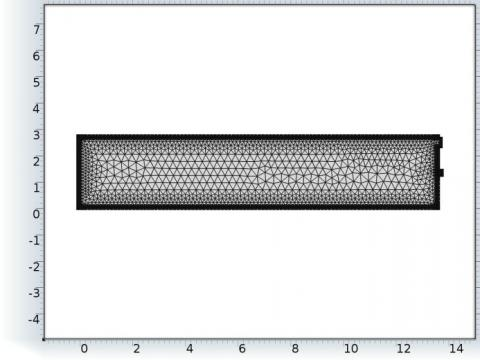Figure 9. Mobil container mesh for laminar regime

The study was conducted in time-dependent mode. It has been set a maximum simulation time, in the case of laminar flow, equal to 2 hours (7200s) with a step of 5 minutes (300s). As last setting the solver more appropriate for this type of application has been defined and the model so configured has been sent running. The running time was approximately 30 minutes with the selected mesh and this simulation has been performed on a personal computer equipped with a processor i5 quad-core 3.1 Ghz.

Figure 10 shows the trend of the average temperature, which is expressed in K, in the whole z-x surface container from the beginning of the simulation up to 2 hours.

As you can see, after a transition, the model tends to reach an average almost constant saturation temperature after the first hour at around -26 °C.

## figure_10.jpg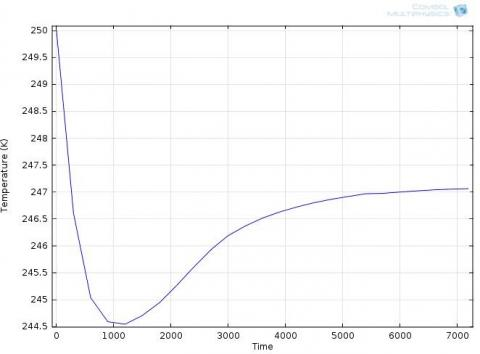Figure 10. Average temperature along z-x surface t = 2h

Therefore Figures 11 and 12 show velocity and temperature fields and in the x-z section container after 60 minutes. You can see for the velocity field the formation of 6 vortices after the first hour which show the trend of the convective flows within the mobile container, and for the temperature field the differences due to the presence of the eutectic plates and of the volume forces.

For this latest simulation we had to make a change of the temperature scale in order to highlight the smallest temperature differences due to convective motions inside container. 6 vortices are visible also here after the first hour and in any case there is a rather uniform temperature inside the container, except near the walls.

## figure_11.jpgFigure 11. Velocity field at t = 60 min

## figure_12.jpg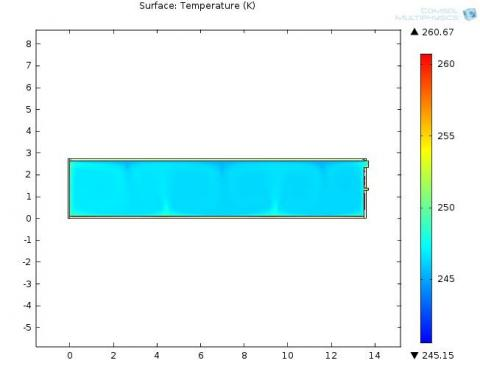Figure 12. Temperature field at t = 60 min

Figure 13 shows the trend of the temperatures along the length x at mid-height of the container, after one hour of the simulation.

## figure_13.png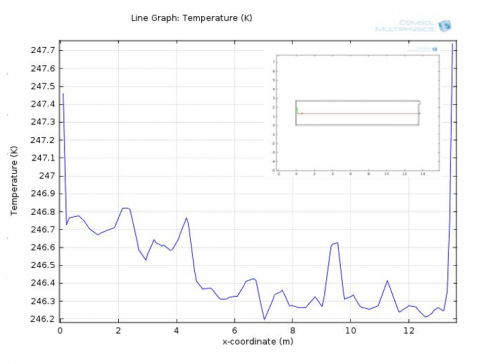Figure 13. Temperature along x axis, z = max/2 and t = 1h

Figures 14-16 show, however, the trend of the temperature to the height varying from the roof to the floor, close to the compressor, near the doors and the center of the container.

## figure_14.png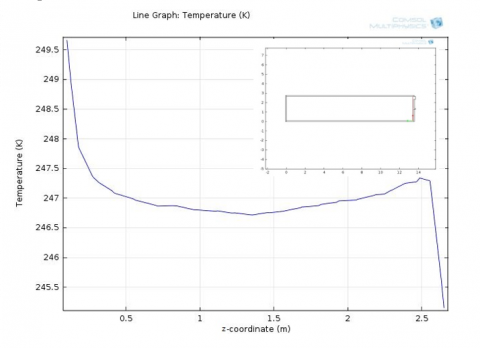Figure 14. Temperature along z axis, x = max and t = 1h

## figure_15.pngFigure 15.  Temperature along z axis, x = 0 e  t = 1h

## figure_16.jpg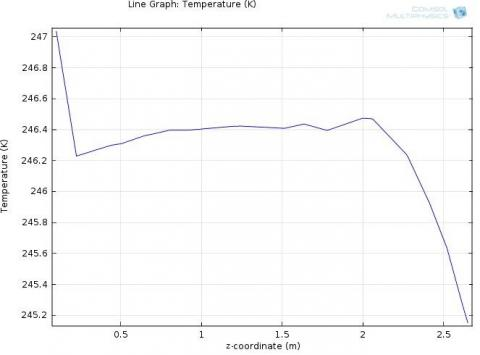Figure 16. Temperature along z axis, x = max/2 e, t = 1h

From these trends of the temperatures along the x axis of the container, after the first hour of the simulation model, it is highlighted that the temperature at half height ranges from -26.7 °C for the central area and compressor area, to -26.2 °C for the rear area; along the z-axis the temperature trend shows that, for the compressor area the temperature ranges from -25.5 °C to -26 °C, for the central area is around -26.6 °C and for the doors area ranges from -25.5 °C to -24.5 °C. Therefore the central area always remains the coldest area in comparison to the other two areas and, in addition, only along the z direction there are temperature differences minor of 1 °C due to the height difference.

3.3 Turbulence flow

It was reproduced an empty container model in turbulent flow to simulate the ON cycle of the compressor.

The materials defined in turbulent flow (figure 8) are the same as laminar flow, the only difference is the boundary 9 of eutectic plates because in turbulent flow with the compressor turned on during the ON phase, the eutectic plates are refrozen and therefore their temperature cannot be considered constant, but decreasing. Therefore their action characterized by a laminar regime, it can be considered negligible compared to that of the compressor in the turbulent regime.

It has been defined a model that uses as resolution technique of the turbulent flows the integration of the Reynolds averaged equations (RANS, Reynolds Averaged Navier Stokes)  . With the different possible turbulence models present in the literature in similar applications and available in Comsol (k-ε, k-ω, ..), some test simulations were made to determine the most suitable turbulence model for the analysis of the convective flows within the mobile container  .

The model chosen has been the k-ε:

$\rho \frac{\partial u}{\partial t}+\rho(u \cdot \nabla) u=\nabla \cdot\left[-p 2 I+\left(\mu+\mu_{T}\right)\left(\nabla u+(\nabla u)^{T}\right)-\frac{2}{3}\left(\mu+\mu_{T}\right)(\nabla \cdot u) I-\frac{2}{3} \rho k 2 I\right]+F$(1)

Starting from left side, the various terms correspond to inertial forces, pressure forces, viscous forces, and volume forces F = -ρ·g. These equations are always resolved with the equation of continuity:

$\frac{\partial \rho}{\partial t}+\nabla \cdot(\rho u)=0$(2)

The Navier-Stokes equation representing the conservation of momentum, while the continuity equation represents the conservation of mass.

In addition there are those relating to the balance for the turbulent kinetic energy k and the dissipation rate per unit mass of ε.

$\rho \frac{\partial k}{\partial t}+\rho(u \cdot \nabla) k=\nabla \cdot\left[\left(\mu+\frac{\mu_{T}}{\sigma_{k}}\right) \nabla k\right]+P_{k}-\rho \varepsilon$(3)

$\rho \frac{\partial \varepsilon}{\partial t}+\rho(u \cdot \nabla) \varepsilon=\nabla \cdot\left[\left(\mu+\frac{\mu_{T}}{\sigma_{\varepsilon}}\right) \nabla \varepsilon\right]+C_{\varepsilon 1} \frac{\varepsilon}{k} P_{k}-C_{\varepsilon 2} \rho \frac{\varepsilon^{2}}{k}$ (4)

dove $\mu_{T}=\rho C_{\mu} \frac{k^{2}}{\varepsilon}$ e $P_{k}=\mu_{T}\left[\nabla u \cdot\left(\nabla u+(\nabla u)^{T}\right)-\frac{2}{3}(\nabla \cdot u)^{2}\right]-\frac{2}{3} \rho k \nabla \cdot u$(5)

Equally to the laminar regime we defined the fluid (Air), the insulating walls (Thermal insulation) and the heat exchange walls (Wall), the initial conditions (Initial values) with the initial temperature of the case T = -22 °C, the conduction of heat through the walls of the case (heat transfer in solid), Volume forces, the initial value of the pressure (Pressure Point Constraint), the outside temperature T = 20° C, the convective flows with the outside (Convective Cooling).

In addition we defined the boundary conditions of inlet and outlet of cold air introduced from the compressor inside the container T = -23°C .

The Reynolds number, defined as Re = ρu0xLT/μ, corresponds to the ratio between the inertial and viscous forces and measure how much turbulent is the flow; with low values flows are laminar, with high values they are turbulent. According to these boundary conditions the Re is equal to about 1.3*104 with ρ and μ, respectively:

μ = 1.6*10-5(kg/ms)

ρ = 0.74 kg/m3

L = 4S/P = 0.3m (hydraulic diameter for rectangular equivalences) to the external operating temperature.

The boundary conditions of inlet Ix defined as Turbulent intensity and LT as Turbulence length scale has been calculated as:

Ix= 0.16 (ReL)-1/8 = 0.03 (3%)

LT = 0.07 L = 0.02 m

u0x = -15 m/s

Ix and LT  values  were used for the calculation of k and ε:

$k_{0}=\frac{3}{2}\left(u_{0 x} / I_{x}\right)^{2} \mathrm{e} \varepsilon_{0}=C_{\mu}^{3 / 4} \frac{k^{3 / 2}}{L_{T}}$(6)

The turbulence model is valid inside the container in all regions of turbulence; in the vicinity of the walls, where the viscous effects are dominant, the model has been used together with the wall functions, boundary conditions of the walls. Also for the turbulent regime the shape of the cells of the identified mesh is triangular and not uniform. Unlike the laminar regime, however, after several test simulations we opted for the extra coarse mesh (Figure 17).

## figure_17.png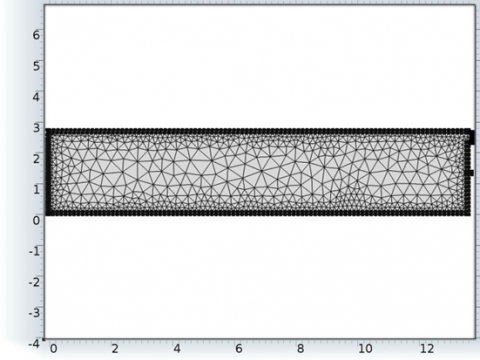Figure 17. Mobil container mesh for turbolent regime

Also in this case the study was conducted in time-dependent way. A maximum simulation time, in the case of turbulent flow, has been set, equal to 4 minutes (240 s) with step of 1 second. This choice has been made in consideration of the execution time (14h for 1 minute simulation) and of the fact that, after 3 minutes, the temperature already reaches values appropriate to the experimental data acquired and additional minutes of execution does not lead to significant changes. Moreover, the construction of the simulation model was induced by the need to verify not the absolute value of the temperature inside of the container, but rather the uniformity of distribution of the cold inside and then the differences that they can generate between different inner areas. As last setting the most appropriate solver was defined for this type of application (Solver) and the so configured model has been sent running. The total run time was about 60 hours with the selected mesh and this simulation was performed on a personal computer equipped with a processor i5 quad-core 3.1 Ghz.

## figure_18.jpg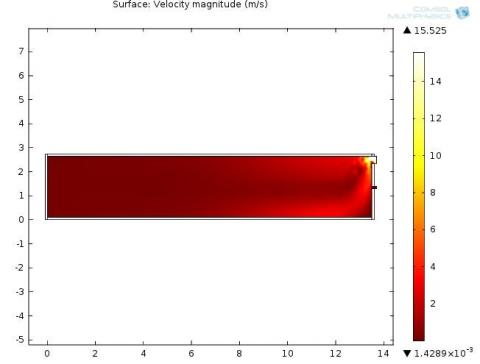Figure 18. Velocity field at t = 3 min

## figure_19.jpg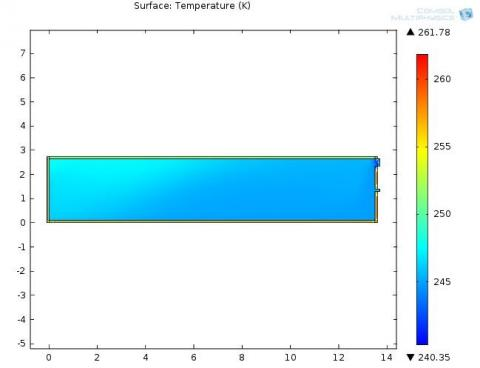Figure 19. Temperature field at t = 3 min

In figures 18-19 there are the fields of velocities and temperatures in the z-x section of the container after 3 minutes. It may be noted for the velocity field the different distribution, in proximity of the compressor inlet, compared to other points within the mobile container, and for the temperature field the differences due to the coldest air introduced from the compressor which gradually distributes itself inside the mobile container. The range of velocities and temperatures are automatically adjusted to the progressive change with the increase of the model execution time.

## figure_20.png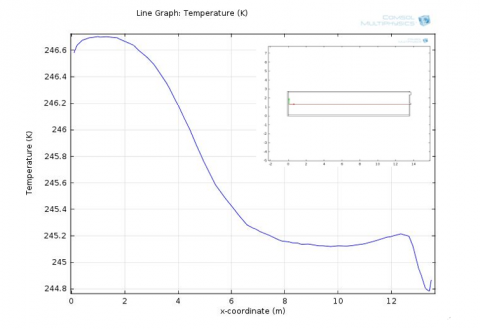Figure 20. Temperature along x axis, z = max/2 and t = 3 min

## figure_21.jpg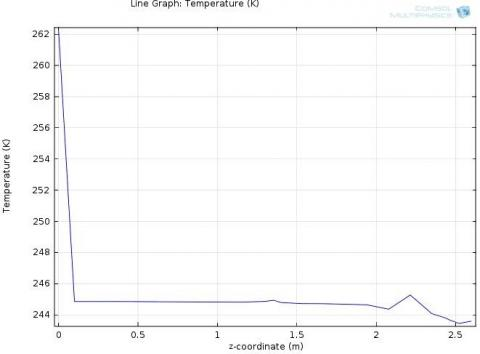Figure 21. Temperature along z axis, x = max and t = 3 min

## figure_22.png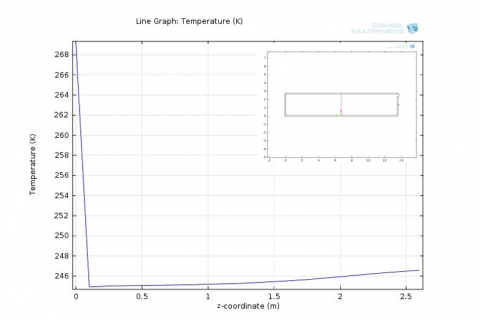Figure 22. Temperature along z axis, x = max/2 and t =3 min

## figure_23.pngFigure 23. Temperature along z axis, x = 0 and t = 3 min

In Figure 20 it is shown the trend of the temperatures along the length of the container at half height after 3 minutes of simulation of the model.

In Figures 21-23 it is shown instead the temperature trend with changes in height from the roof to the floor, close to the compressor, in the center of container and close to the doors. From these trends of the temperatures along the x axis of the container, after 3 minutes of the simulation model for the turbulent regime, is evident that at mid-height the temperature goes from -27.8 °C for the central area and the area near the compressor to -26.4 °C for the rear area.

Along the z-axis the trend of temperatures is almost constant or with differences of 1 °C. For the compressor area the temperature is around -28 °C, for the central one the temperature ranges from -27 °C under the roof to -27.5 °C on the floor and for the doors area from 26 °C under the roof to -27 °C on the floor, so the area close to the compressor is clearly colder than the other two areas, because of the distance of the cold air inlet point from the bottom of the container; anyway the difference between the compressor area and the doors area reaches a maximum of 2 °C.

4. Comparison Between Experimental and Numerical Model

After the development of the model by Comsol and once obtained the results, we have validate them in order to give information about the quality of the model. Despite the limited number of probes installed in the container, the data acquired during experimentation were adequately compared with the experimental ones processed by the model .

4.1 Laminar flow

To compare the experimental data recorded by PT100 positioned at mid-height of the container with data simulated by the model in Comsol, we considered the temperature trend along the x axis at height of zmax/2 (Figure 24).

## figure_24.png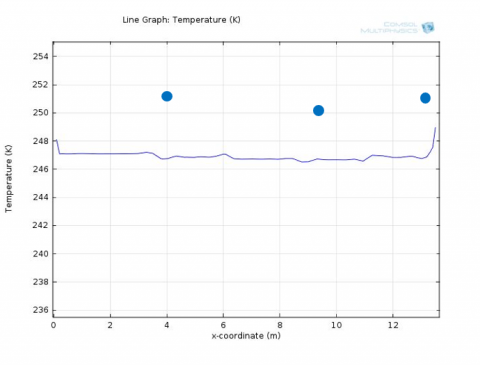Figure 24. Comparison between numerical and experimental temperature data

In the model, after the first hour of simulation, it has been considered as time frame Δt = 20 minutes, corresponding to the ON-OFF range of the probes acquisitions, to allow the model to achieve regime values. A deviation is found, equal to approximately 3 °C between experimental and simulated data, absolutely acceptable due to the simplifying assumptions useful for the definition of the developed model. However the temperature differences in the different thermal areas of the container are respected, because the central area is slightly cooler than the other two neighboring areas both in the experimentation that in the model.

4.2 Turbulence flow

## figure_25.png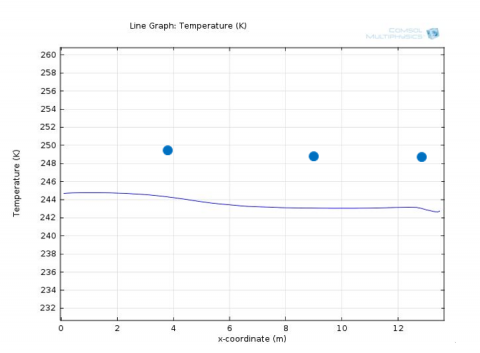Figure 25. Comparison between numerical and experimental temperature data

To compare the experimental data recorded by PT100 probes positioned at half height of the container with data simulated by the model in Comsol for the turbulent regime, we considered the temperature trend along the x axis with a height of zmax/2 (Figures 25). As time frame in the simulation model it has been considered a Δt = 4 minutes, because after this time the temperatures reach these values and, considering also the high execution times, the simulation model does not introduce more information. The model doesn’t try to verify the absolute values of temperatures in various points inside of the container, but the uniformity of cold distribution along the various directions of the container. Therefore a deviation, equal to about 4-5 °C, is found between experimental and simulated data, also in this case totally acceptable due to the simplifying assumptions made for model definition. However, the observed temperature differences between the area close to the compressor and the other two neighboring areas are clearly due to the position of the inlet point of cold air inside the mobile container.

5. Conclusions

The goal numerical and experimental analysis of convective flows inside the container has been to check the uniformity of cold distribution . Therefore we have tried to get, through the software Comsol, the trends of convective flows related to velocity and temperature. The comparison between experimental data acquired during the simulated trip and processed numerical data allowed the validation of the implemented model. Because of the considerable container dimensions, a greater number of equally spaced temperature probes and also additional information regarding the distribution of air velocity inside the container, would have allowed the development of a more accurate model.

As regards the laminar regime, there is a slight deviation in absolute values between experimental and simulated data, due to the initial simplifying assumptions. However, both data show the same minimum temperature differences in the thermal areas of the container, so the central one is slightly cooler than the other two neighboring areas.

Regarding the turbulent regime, it is confirmed the deviation between experimental and simulated data. The temperature differences observed between the area close to the compressor and the other two neighboring areas are clearly due to the position of the inlet point of cold air inside the mobile container. Due to the long execution time, and considering that more minutes does not lead to significant changes in temperature values, a reduced simulation interval was chosen than the actual cycle of the compressor switch-on.

The application of the modeling techniques of convective flows to refrigeration systems, so provides an opportunity to improve the knowledge of the phenomena, in order to reduce the heterogeneity of the distribution of temperature and increase the efficiency of such refrigeration systems.

Nomenclature
 CP specific heat, J. kg-1. K-1 Cµ,Cε1,Cε2,σε,σk I L LT p turbulence model coefficients turbulent intensity, % hydraulic diameter for rectangular equivalence of the inlet section, m turbulence length scale, m static pressure, Pa Q Re flow rate, m3. s-1 Reynolds number T temperature, K u velocity, m-1. s-1 Greek symbols ρ density  kg. m-3 k kinetic energy of turbulence m2. s-2 ε turbulence energy dissipation rate m2.s-3 µ dynamic viscosity, kg. m-1.s-1 Subscripts T turbulent x vector direction
References

 S. Estrada-Flores and A. Eddy, “Thermal performance indicators for refrigerated road vehicles,” Int J of Refrigeration, vol. 29, no. 6, pp. 889-898, 2006. DOI:  10.1016/j.ijrefrig.2006.01.012.

 http://www.comsol.com/multiphysics

 Qianjian Guo, Xiaoni Qi, Zheng Wei, Pengjiang Guo and Peng Sun, “3D Numerical simulation and analysis of refrigeration performance of the small diameter vortex tube,” International Journal of Heat and Technology, vol. 34 no. 3, pp. 513-520, 2016. DOI: 10.18280/ijht.340324.

 P. Gardin, M. Brunet, Domgin J. F. and  K. Pericleous, “An experimental and numerical CFD study of turbulence in a tundish container,” Applied Mathematical Modelling, vol. 26, no. 2, pp. 323-336,  2002. DOI: 10.1016/S0307-904X(01)00064-6.

 D. Kaliakatsos, M. Cucumo, V. Ferraro, M. Mele, A. Galloro and F. Accorinti. “CFD Analysis of a Pipe equipped with twisted tape,” International Journal of Heat and Technology, vol. 34, no. 2, pp.172-180, 2016. DOI: 10.18280/ijht.340203.

 J. Moureh and D. Flick, “Airflow pattern and temperature distribution in a typical refrigerated truck configuration loaded with pallets,” Int J of Refrigeration, vol. 27, no. 5, pp. 464-474, 2004. DOI: 10.1016/j.ijrefrig.2004.03.003.

 N. J. Smale, J. Moureh and G. Cortella, “A review of numerical models of airflow in refrigerated food applications,” Int J of Refrigeration, vol. 29, no. 6, pp. 911-930, 2006. DOI: 10.1016/j.ijrefrig.2006.03.019.

 H. S. Yun, J. K. Kwon, H. D. Lee, Y. K. Kim and N. K.  Yun, “CFD Simulation of airflow and heat transfer in the cold container,” J of Biosystems Eng., vol. 32, no. 6, pp. 422-429, 2007.

 B. E. Launder and D. B Spalding, “The numerical computation of turbulence flows,” Comput Methods Appl Mech Energy, vol. 3, pp. 269-89, 1974.

 J. Rodriguez-Bermejo, P. Barreiro, J. I. Robla and L.  Ruiz-Garcia, “Thermal study of a transport container,” J of Food Eng, vol. 80, no. 2, pp. 517-527, 2007. DOI:  10.1016/j.jfoodeng.2006.06.010.